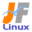Linux Kernel 2.2 Documentation: /usr/src/linux/Documentation/scsi.txt

scsi.txt

Linux \$B%+!<%M%k\$K\$*\$1\$k(B SCSI \$B\$N%5%]!<%H(B [\$B%W%l%\$%s%F%-%9%HHG(B]
• \$B86Cx:n
• \$BK]Lu
• \$B%P!<%8%g%s(B: 2.2.0
• \$BK]LuF|;~(B: 1997/06/27
```

The scsi support in the linux kernel can be modularized in a
number of different ways depending upon the needs of the end user.  To
understand  your options, we should first define a few terms.

Linux \$B%+!<%M%k\$K\$*\$1\$k(B SCSI \$B\$N%5%]!<%H\$O!\$%(%s%I%f!<%6\$NMW7o\$K\$h\$j\$N(B SCSI \$B%I%i%\$%P(B
\$B\$rMxMQ\$9\$k\$3\$H\$O\$G\$-\$^\$;\$s!%(Bscsi-core \$B\$O%b%8%e!<%k(B (scsi_mod.o) \$B\$K\$G\$-(B
\$B\$^\$9\$7!\$%+!<%M%kFb\$KAH\$_9~\$`\$3\$H\$b\$G\$-\$^\$9!%%b%8%e!<%k\$K\$7\$?>l9g\$O!\$(B
SCSI \$B4X78\$N%b%8%e!<%k\$N\$&\$A\$G:G=i\$K%m!<%I\$5\$l\$M\$P\$J\$j\$^\$;\$s\$7!\$%"%s%m!<(B
\$B%I\$O:G8e\$K\$J\$5\$l\$M\$P\$J\$j\$^\$;\$s!%(B

The individual upper and lower level drivers can be loaded in any
order once the scsi core is present in the kernel (either compiled in
or loaded as a module).  The disk driver (sd_mod.o), cdrom driver (sr_mod.o),
tape driver (st.o) and scsi generics driver (sg.o) represent the upper level
drivers to support the various assorted devices which can be controlled.
You can for example load the tape driver to use the tape drive, and then
unload it once you have no further need for the driver (and release the
associated memory).

\$B8D!9\$N>e0L%l%Y%k%I%i%\$%P\$d2<0L%l%Y%k%I%i%\$%P\$O!\$(Bscsi core \$B\$,%+!<%M%k(B
\$BFb\$K%m!<%I!J%+!<%M%k\$KAH\$_9~\$_\$^\$?\$O%b%8%e!<%k\$H\$7\$F%m!<%I!K\$5\$l\$?8e\$G(B
\$B\$"\$l\$P!\$\$I\$N\$h\$&\$J=g=x\$G\$b%m!<%I\$G\$-\$^\$9!%%G%#%9%/%I%i%\$%P(B (sd_mod.o)
\$B\$d(B CDROM \$B%I%i%\$%P(B(sr_mod.o) \$B!\$%F!<%W%I%i%\$%P(B (st.o) \$B!\$HFMQ(B SCSI \$B%I%i%\$(B
\$B%P(B (sg.o) \$B\$O!\$BP1~\$9\$ke0L%l%Y%k%I%i%\$%P\$rI=\$7(B
\$B\$F\$\$\$^\$9!%Nc\$(\$P!\$%F!<%WAuCV\$rMxMQ\$9\$k\$K\$O%F!<%W%I%i%\$%P\$r%m!<%I\$7!\$%I(B
\$B%i%\$%P\$,I,MW\$J\$/\$J\$l\$P%"%s%m!<%I\$G\$-\$^\$9!J\$=\$7\$F4XO"\$9\$k%a%b%j\$r2rJ|\$7(B
\$B\$^\$9!K!%(B

The lower level drivers are the ones that support the
individual cards that are supported for the hardware platform that you
are running under.  Examples are aha1542.o to drive Adaptec 1542
cards.  Rather than list the drivers which *can* be modularized, it is
easier to list the ones which cannot, since the list only contains a
few entries.  The drivers which have NOT been modularized are:

NCR5380 boards of one kind or another including PAS16,
Trantor T128/128F/228,

\$B2<0L%l%Y%k%I%i%\$%P\$H\$O!\$;HMQ\$9\$k%O!<%I%&%'%"%W%i%C%H%U%)!<%`\$KBP\$7\$F(B